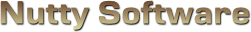Sorry, it appears you don't have support for WebGL.

In order to run this demo, you must meet the following requirements.

• You have a WebGL compatible video card with the latest drivers.
• Your video card is not blacklisted. You can check the current blacklist on Khronos.

Some browsers may require additional configuration in order to get WebGL to run. If you are having problems running this demo, visit the following sites.

#### Vignetting

					
/// <summary>
/// Attributes.
/// <summary>
attribute vec3 Vertex;
attribute vec2 Uv;

/// <summary>
/// Uniform variables.
/// <summary>
uniform mat4 ProjectionMatrix;
uniform mat4 ViewMatrix;
uniform mat4 ModelMatrix;
uniform vec3 ModelScale;

/// <summary>
/// Varying variables.
/// <summary>
varying vec2 vUv;

/// <summary>
/// <summary>
void main ()
{
vec4 worldVertex = ModelMatrix * vec4(Vertex * ModelScale, 1.0);
vec4 viewVertex = ViewMatrix * worldVertex;
gl_Position = ProjectionMatrix * viewVertex;

vUv = Uv;
}


					
#ifdef GL_ES
precision highp float;
#endif

/// <summary>
/// Uniform variables.
/// <summary>
uniform vec2 ImageSize;
uniform vec2 TexelSize;
uniform vec4 Colour;
uniform sampler2D Sample0;

uniform float SepiaValue;
uniform float NoiseValue;
uniform float ScratchValue;
uniform float InnerVignetting;
uniform float OuterVignetting;
uniform float RandomValue;
uniform float TimeLapse;

/// <summary>
/// Varying variables.
/// <summary>
varying vec2 vUv;

/// <summary>
/// Computes the overlay between the source and destination colours.
/// <summary>
vec3 Overlay (vec3 src, vec3 dst)
{
// if (dst <= �) then: 2 * src * dst
// if (dst > �) then: 1 - 2 * (1 - dst) * (1 - src)
return vec3((dst.x <= 0.5) ? (2.0 * src.x * dst.x) : (1.0 - 2.0 * (1.0 - dst.x) * (1.0 - src.x)),
(dst.y <= 0.5) ? (2.0 * src.y * dst.y) : (1.0 - 2.0 * (1.0 - dst.y) * (1.0 - src.y)),
(dst.z <= 0.5) ? (2.0 * src.z * dst.z) : (1.0 - 2.0 * (1.0 - dst.z) * (1.0 - src.z)));
}

/// <summary>
/// 2D Noise by Ian McEwan, Ashima Arts.
/// <summary>
vec3 mod289(vec3 x) { return x - floor(x * (1.0 / 289.0)) * 289.0; }
vec2 mod289(vec2 x) { return x - floor(x * (1.0 / 289.0)) * 289.0; }
vec3 permute(vec3 x) { return mod289(((x*34.0)+1.0)*x); }
float snoise (vec2 v)
{
const vec4 C = vec4(0.211324865405187,	// (3.0-sqrt(3.0))/6.0
0.366025403784439,	// 0.5*(sqrt(3.0)-1.0)
-0.577350269189626,	// -1.0 + 2.0 * C.x
0.024390243902439);	// 1.0 / 41.0

// First corner
vec2 i  = floor(v + dot(v, C.yy) );
vec2 x0 = v -   i + dot(i, C.xx);

// Other corners
vec2 i1;
i1 = (x0.x > x0.y) ? vec2(1.0, 0.0) : vec2(0.0, 1.0);
vec4 x12 = x0.xyxy + C.xxzz;
x12.xy -= i1;

// Permutations
i = mod289(i); // Avoid truncation effects in permutation
vec3 p = permute( permute( i.y + vec3(0.0, i1.y, 1.0 ))
+ i.x + vec3(0.0, i1.x, 1.0 ));

vec3 m = max(0.5 - vec3(dot(x0,x0), dot(x12.xy,x12.xy), dot(x12.zw,x12.zw)), 0.0);
m = m*m ;
m = m*m ;

// Gradients: 41 points uniformly over a line, mapped onto a diamond.
// The ring size 17*17 = 289 is close to a multiple of 41 (41*7 = 287)

vec3 x = 2.0 * fract(p * C.www) - 1.0;
vec3 h = abs(x) - 0.5;
vec3 ox = floor(x + 0.5);
vec3 a0 = x - ox;

// Normalise gradients implicitly by scaling m
// Approximation of: m *= inversesqrt( a0*a0 + h*h );
m *= 1.79284291400159 - 0.85373472095314 * ( a0*a0 + h*h );

// Compute final noise value at P
vec3 g;
g.x  = a0.x  * x0.x  + h.x  * x0.y;
g.yz = a0.yz * x12.xz + h.yz * x12.yw;
return 130.0 * dot(m, g);
}

/// <summary>
/// <summary>
void main ()
{
// Sepia RGB value
vec3 sepia = vec3(112.0 / 255.0, 66.0 / 255.0, 20.0 / 255.0);

// Step 1: Convert to grayscale
vec3 colour = texture2D(Sample0, vUv).xyz;
float gray = (colour.x + colour.y + colour.z) / 3.0;
vec3 grayscale = vec3(gray);

// Step 2: Appy sepia overlay
vec3 finalColour = Overlay(sepia, grayscale);

// Step 3: Lerp final sepia colour
finalColour = grayscale + SepiaValue * (finalColour - grayscale);

float noise = snoise(vUv * vec2(1024.0 + RandomValue * 512.0, 1024.0 + RandomValue * 512.0)) * 0.5;
finalColour += noise * NoiseValue;

// Optionally add noise as an overlay, simulating ISO on the camera
//vec3 noiseOverlay = Overlay(finalColour, vec3(noise));
//finalColour = finalColour + NoiseValue * (finalColour - noiseOverlay);

// Step 5: Apply scratches
if ( RandomValue < ScratchValue )
{
// Pick a random spot to show scratches
float dist = 1.0 / ScratchValue;
float d = distance(vUv, vec2(RandomValue * dist, RandomValue * dist));
if ( d < 0.4 )
{
// Generate the scratch
float xPeriod = 8.0;
float yPeriod = 1.0;
float pi = 3.141592;
float phase = TimeLapse;
float turbulence = snoise(vUv * 2.5);
float vScratch = 0.5 + (sin(((vUv.x * xPeriod + vUv.y * yPeriod + turbulence)) * pi + phase) * 0.5);
vScratch = clamp((vScratch * 10000.0) + 0.35, 0.0, 1.0);

finalColour.xyz *= vScratch;
}
}

// Step 6: Apply vignetting
// Max distance from centre to corner is ~0.7. Scale that to 1.0.
float d = distance(vec2(0.5, 0.5), vUv) * 1.414213;
float vignetting = clamp((OuterVignetting - d) / (OuterVignetting - InnerVignetting), 0.0, 1.0);
finalColour.xyz *= vignetting;

// Apply colour
gl_FragColor.xyz = finalColour;
gl_FragColor.w = 1.0;
}



# Old Film Effect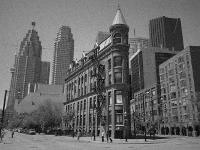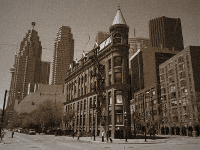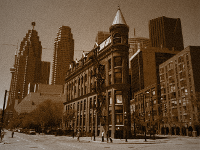## Abstract

The purpose of this shader is to demonstrate how you can use sepia toning, noise, film scratches, and vignetting to produce a classic looking film effect. This is a post-process effect, so you render your scene normally to a texture using a fragment buffer object and then operate on that rendered image in a second pass using this shader. The controls in the WebGL demo allow you to manipulate the percentages of each effect, showing you how each effect contributes to the final image.

## History

Over the past century and a half, much research and work has been done to improve the quality, performance, and longevity of film. In the earliest days of photography, various techniques were used to process photographs. Common in all techniques was the use of silver, which has interesting photosensitive properties when mixed with other chemicals. This birthed the commercialization of black and white photography, which even today remains a popular choice for certain photographs. While a large portion of classic photographs were processed black and white, an added chemical process was commonly used to improve the longevity of a photograph. This was known as sepia treatment, which is named after the Sepia cuttlefish that produces the chemical. Much of the old photographs you see today in such good condition are attributed to this process.

## Sepia Toning

In the early days of photography, all developed photographs were black and white. Sepia treatment was an added chemical process that converted the silver in the photograph to a sulfide, which not only improves the longevity of the photograph, but also accounts for the brownish tone. This can be simulated in software using a duotone algorithm.

The first step is to convert the image into grayscale. One method to convert an image to grayscale is to calculate the average of your colour channels. An RGB image for example could be converted to grayscale using the following formula.

$I = (C_R + C_G + C_B) / 3$

Where

$C_R$ is the red component value.

$C_G$ is the green component value.

$C_B$ is the blue component value.

$I$ is the average intensity value of the RGB colour.

Once you produce a grayscale image, the next step is to apply the sepia tone. Sepia is the name of a colour, just like blue and green. It has the RGB value (112, 66, 20), or hexidecimal value #704214. The idea is to blend this colour value with the rendered grayscale image. There are several image blending techniques that can be used to produce this effect and each blending technique has a different final result. This shader uses an overlay blending algorithm. An overlay is similar to placing a translucent film on top of an object, which causes the original colour to morph into the colour of the overlay. This gives you the following result.+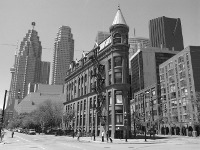=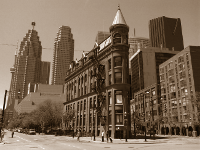The formula for the overlay blend operation is described below.

$F_{RGB} = \left\{ \begin{matrix} 2.0 * S_{RGB} * D_{RGB} & D_{RGB} \leq 0.5 \\ 1.0 - 2.0 * (1.0 - D_{RGB}) * (1.0 - S_{RGB}) & D_{RGB} > 0.5 \end{matrix} \right\}$

Where

$S_{RGB}$ is the source colour.

$D_{RGB}$ is the destination colour.

$F_{RGB}$ is the final colour.

There are two formulae to chose from. The one you use depends on the destination component value. When computing the final red component for example, you have to check if the destination red component is less than or greater than 0.5 to determine which formula you use. In the case with a grayscale image, you use the gray value. This formula assumes your colour channels are stored in floating point format. That is, each colour is within the range 0.0 to 1.0.

In the example above, the sepia colour is the source image and the grayscale rendering is the destination image. The ordering of these images matter because if the grayscale image was made the source and the sepia colour the destination, then you would be calculating what's called “Hard Lighting”. This is another type of blending technique that produces a different look.

## Noise

Computer graphics are rendered using perfect algorithms. That is to say, computer graphics don't have to suffer the same physical constraints as real hardware does. They don't suffer from film grain, lens flare, chromatic aberration, etc. These effects have to be simulated in computer graphics by programming them into the render pipeline. Noise is a great way to simulate film grain, which is a result of a film or digital camera generating noise due to amplifying the luminosity (or signal), usually as a result of insufficient lighting to illuminate the subject. To calculate noise, the shader uses an efficient 2D simplex noise algorithm implemented by Ian McEwan from Ashima Arts. The result of adding noise to the image is illustrated below.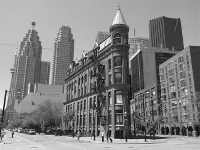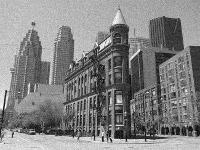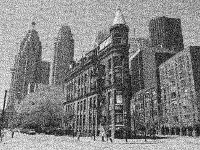The image on the left has no noise, which appears plain. The image in the middle introduces a little noise and the image on the right demonstrates extreme noise. The amount of noise you apply to a scene can vary, but even a little amount of noise adds detail and simulates a realistic camera.

The simplex noise algorithm is not discussed here. If you would like to learn more about Simplex noise, take a look at Stefan Gustavson's PDF document explaining the algorithm. You can also lookup the original Perlin noise algorithm, which simplex noise is based off.

## Scratches

Scratches are signs of wear and tear on film. It can be created as a result of poor film quality, cleaning film with a rough surface, exposure to the elements, or poor handling when the film is being processed or edited. These appear as randomly occurring thin lines throughout the video. The effect can simulated in software using a turbulent sine algorithm. The effect is illustrated below.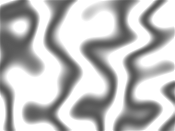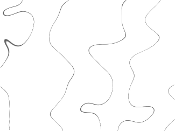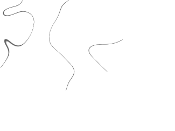The first image shows a series of sine bands, which are generated using the sine function.

$S_{band} = � + (sin(((T_X * UV_X) + (T_Y * UV_Y)) * 2 \pi + \phi) * �)$

Where

$T_X$is the period along the x-axis. This controls how many vertical bands are generated.

$T_Y$is the period along the y-axis. This controls how must horizontal bands are generated, or tilt.

$\phi$is the phase value, for shifting the sine wave.

Both Tx and Ty are multiplied by the UV coordinates of the image. In the above example, there are 4 bands along the horizontal axis. To simulate this effect, you would supply a Tx value of 4.0. The bands are slightly titled due to using a Ty period of about 1.0. By multiplying these two by the corresonding UV coordinates, you generate an image with the illustrated bands. Since the sine function produces a value -1.0 <= x <= 1.0, you need to clamp it to the proper colour range of 0.0 <=x <= 1.0. To do this, the calculated sine value is multiplied by ½ and then added to ½.

The second image adds a turbulent factor to the sine function. The turbulent value is extracted from the 2D simplex noise algorithm using the current UV coordinate and some multiplier. This changes the formula to:

$S_{band} = � + (sin(((T_X * UV_X) + (T_Y * UV_Y) + Turbulence) * 2 \pi + \phi) * �)$

Where

Turbulence = noise2D(UV * multiplier)

multiplier is an arbitrary value that increases or decreases the turbulent factor. The above image used a multiplier of 2.5.

The third image shows what happens when you increase the intensity 10000 fold. Only the darkest cracks in the sine bands remain dark. These will be used to simulate the film scratches, but it's not a good idea to show scratches for the entire frame. Usually only select regions display any sort of scratching, which is demonstrated in the fourth image. To simulate this effect, you randomly pick a point on the image and show only the scratches produced within that area. This is done using the distance formula.

$d = distance(X_{rand}, Y_{rand}) \left\{ \begin{matrix} 0.0 \leq X_{rand} \leq 1.0 \\ 0.0 \leq Y_{rand} \leq 1.0 \end{matrix} \right\}$

This formula takes as input a random location on the image. If the calculated distance is less then some value, say 0.4 for example, then you can proceed to calculate any scratches in that region. The result is then multiplied onto the final image.

## Vignetting

The final piece to the puzzle is vignetting. Vignetting is the dimming or complete occlusion of light on the captured frame due primarily to an improperly fitted lens hood on the camera. Sometimes this is an intentional effect whereby the director wants to put focus on the subject in the centre of the frame. This was a common effect in classic films with facial closeups of an actor or actress. To simulate this effect, all you need to do is calculate the distance from the centre of the frame and apply some dimming modifier based on that distance. The further away from the centre, the more dimming you apply. The following demonstrates the circular vignetting zones on the frame.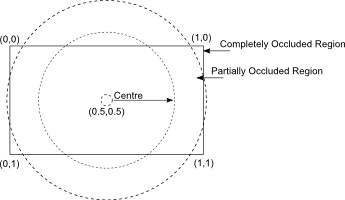Illustration of the circular vignetting effect

The inner circle represents the region untouched by vignetting. The region between the inner and outer circle represent the area where vignetting starts to take place, which is a gradual fade to black from the inner to outer ring. Any part of the frame outside of the outer ring would be completely black. In a fragment shader, the image you are post-processing will have UV coordinates between 0.0 and 1.0, as shown in the illustration. The centre of this image would have the UV value (0.5, 0.5). Using the distance formula, we can calculate the maximum distance from the centre of the frame to any one of its four corners.

$d^2 = u^2 + v^2$

$d^2 = 0.5^2 + 0.5^2$

$d = \sqrt{0.5^2 + 0.5^2}$

$d = \sqrt{0.25 + 0.25}$

$d = 0.707106$

Since UV values fall in the range 0.0 to 1.0, the distance should be scaled to fit the range 0.0 to 1.0. This can be done by multiplying the distance by approximately 1.41, which you will see in the shader code. All that is left now is to calculate the dimming effect based on the distance and the two vignetting rings. This is similar to the formula used for spotlights.

$V = clamp((V_O - d) / (V_O - V_I), 0.0, 1.0)$

Where

$V_O$ is the outer vignetting ring.

$V_I$ is the inner vignetting ring.

$d$ is the distance from the current UV coordinate to the centre of the frame.

$V$ is the calculated vignetting multiplier, clamped to the range 0.0 and 1.0.

After you calculate V, simply multiply this value onto your final fragment colour.

## Enhancements

There are other effects you can apply to the shader to improve realism. One feature left out is camera shake. Old films didn't have the technology cinematographers have today to stabilize the camera during filming. Using the random value passed into the shader, you could offset the camera a tiny bit to simulate a camera shake. Care should be taken only to move the camera fewer than 2 or 3 times per second to avoid an undesirable amount of shaking.

Not all old films have to apply a sepia overlay. You could replace the grayscale and sepia combination with a colour saturation effect to simulate technicolor or its predecessor kinemacolor. You could also replace sepia with another colour, such as blue. By using different colours, you can change the mood of the image.

## References

1. Wikipedia Editors (2011-01-19). “Photography”. Wikipedia. Retrieved 2012-02-19.

2. Photography Editors. “How To Use Sepia Toning”. Photography. Retrieved 2012-02-19.

3. Wikipedia Editors (2012-01-13). “Sepia tone”. Wikipedia. Retrieved 2012-02-19.

The source code for this project is made freely available for download. The ZIP package below contains both the HTML and JavaScript files to replicate this WebGL demo.

The source code utilizes the Nutty Open WebGL Framework, which is an open sourced, simplified version of the closed source Nutty WebGL Framework. It is released under a modified MIT license, so you are free to use if for personal and commercial purposes.